Next: Equivalent Circuits Up: Kirchoff's Laws Previous: Branch Current Method

## Loop Current Method

This method is also referred to as the mesh loop method. The independent current variables are taken to be the circulating current in each of the interior loops.

1. Label interior loop currents on a diagram.
2. Obtain expressions for the voltage changes around each interior loop.
3. Solve the system of algebraic equations.

Depending on the problem, it may ultimately be necessary to algebraically sum two loop currents in order to obtain the needed interior branch current for the final answer.

Lets consider the example of the Wheatstone bridge circuit shown in figure 1.6. We wish to calculate the currents around the loops. The three currents are identified as: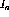the clockwise current around the large interior loop which includes the EMF,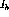the clockwise current around the top equilateral triangle, and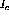the clockwise current around the bottom equilateral triangle. The voltage loop expressions for the three current loops are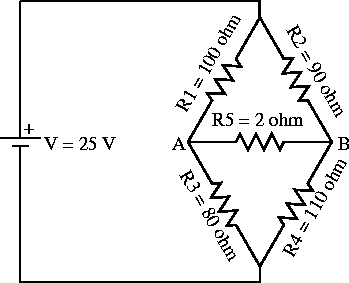Figure 1.6:   Loop method for the Wheatstone bridge circuit.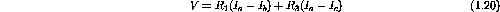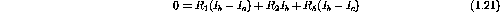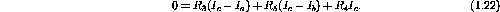Collecting terms containing the same current gives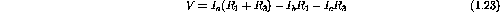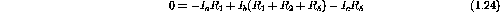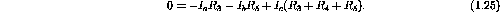If the values for the parameters shown in the diagram are used, the current values can be found by solving the set of simultaneous equations to give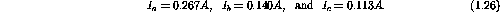Moreover, if we number the individual currents through each resistor using the same scheme as we have for each component (current through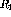is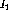,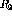has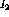, etc.) and identify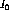as the current out of the battery, then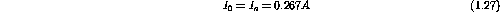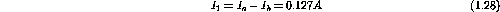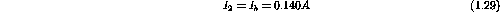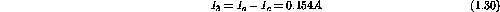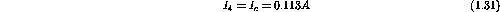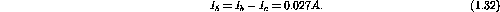These are the same currents that would be found using only Kirchoff's equations; however, here we had to handle only three simultaneous equations instead of six.

Example: Use the loop current method to determine the voltage developed across the terminals AB in the circuit shown in figure 1.7.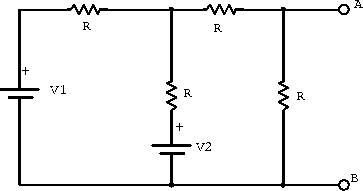Figure 1.7:  Example circuit for analysis using the loop current method.

Consider the clockwise current loop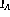through the two resistors and the two potentials. Similarly consider the clockwise current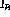around the other internal loop consisting of the three resistors and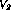. Kirchoff's law gives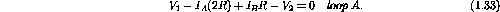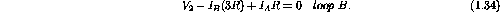Solving the above two equations for the unknown loop currentsandgives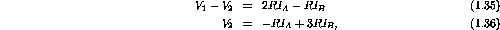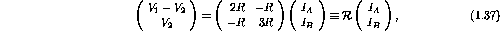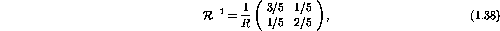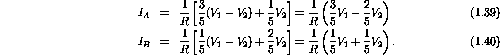The voltage across AB is given simply by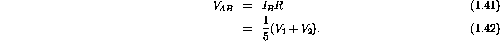Next: Equivalent Circuits Up: Kirchoff's Laws Previous: Branch Current Method

Doug Gingrich
Tue Jul 13 16:55:15 EDT 1999